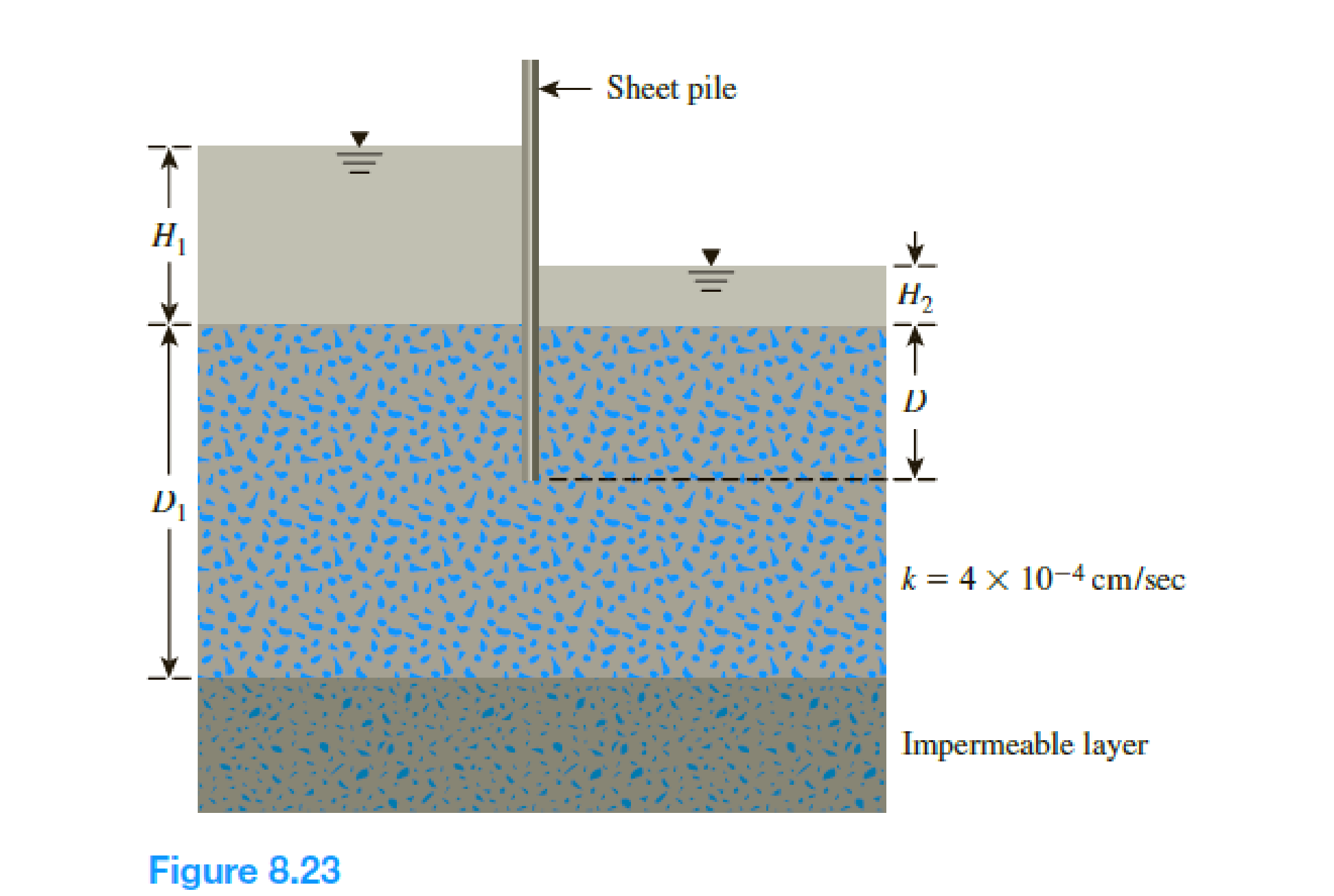Chapter 8, Problem 8.2PPrinciples of Geotechnical Enginee...

9th Edition
Braja M. Das + 1 other
ISBN: 9781305970939

Solutions

Chapter
SectionPrinciples of Geotechnical Enginee...

9th Edition
Braja M. Das + 1 other
ISBN: 9781305970939
Textbook Problem

Draw a flow net for the single row of sheet piles driven into a permeable layer as shown in Figure 8.23. Given: • H1 = 3 m • H2 = 0.5 m • D = 1.5 m • D1 = 3.75 m Calculate the seepage loss per meter length of the sheet pile (at right angles to the cross section shown).To determine

Draw the flow net and calculate the seepage loss per meter length of the sheet pile.

Explanation

Given information:

The hydraulic conductivity of the permeable soil layer k is 4×104cm/sec.

The height of the water level H1 is 3 m.

The height of the water level H2 is 0.5 m.

The depth of permeable layer up to the end of sheet pile D is 1.5 m.

The depth of permeable layer D1 is 3.75 m.

Calculation:

Draw the free body diagram of the flow net for the given values as in Figure 1.

Refer Figure 1.

Determine the head difference between the upstream and downstream using the relation.

H=H1H2

Substitute 3 m for H1 and 0.5 m for H2.

H=30.5=2.5m

Determine the seepage loss per meter length of the sheet pile using the relation.

q=kHNfNd

Here, Nf is the number of flow lines and Nd is the number of potential drops

Still sussing out bartleby?

Check out a sample textbook solution.

See a sample solution

The Solution to Your Study Problems

Bartleby provides explanations to thousands of textbook problems written by our experts, many with advanced degrees!

Get Started

Which type of lighting system has the highest CRI?

Engineering Fundamentals: An Introduction to Engineering (MindTap Course List)

What are the basic characteristics of a NoSQL database?

Database Systems: Design, Implementation, & Management

What is DcepFace and how is it used?

Fundamentals of Information Systems

What are vulnerabilities? How do you identify them?

Principles of Information Security (MindTap Course List)

What does SDS stand for, and what is the purpose of SDSs?

Precision Machining Technology (MindTap Course List)

List the sections of a system design specification, and describe the contents.

Systems Analysis and Design (Shelly Cashman Series) (MindTap Course List)

Describe the activities of the analysis phase. List the three activities of the detailed analysis phase.

Enhanced Discovering Computers 2017 (Shelly Cashman Series) (MindTap Course List)